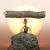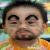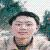## 关于函数指针赋值的问题

pcxsvl 发布于 2014/06/23 11:29

int fn1(int n,int b);

int(*P)(int n,int b);

int a;

p=fn1;

p(3,4);

a=*P;

01、a=p(3,4);  //跟普通函数调用时一样的

2、a=(*p)(3,4)

```#include<iostream>
using namespace std;
int sum(int x, int y){
return x+y;
}
int main(){
int (* funp)(int,int);
funp = sum;
int res1 = funp(3,4);
int res2 = (*funp)(2,4);
cout<<"""res1 = "<<res1<<"  res2 = "<<res2<<endl;
return 0;
}```

0